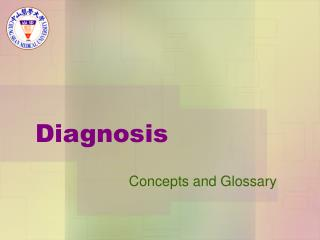DownloadDownload PresentationDiagnosis

# Diagnosis

Télécharger la présentation## Diagnosis

- - - - - - - - - - - - - - - - - - - - - - - - - - - E N D - - - - - - - - - - - - - - - - - - - - - - - - - - -
##### Presentation Transcript

1. Diagnosis Concepts and Glossary

2. Cross-sectional study The observation of a defined population at a single point in time or time interval. Exposure and outcome are determined simultaneously.

3. Sensitivity • Proportion of people with the target disorder who have a positive test. • It is used to assist in assessing and selecting a diagnostic test/sign/symptom.

4. Specificity • Proportion of people without the target disorder who have a negative test. • It is used to assist in assessing and selecting a diagnostic test/sign/symptom.

5. Likelihood ratio (LR) • The likelihood that a given test result would be expected in a patient with the target disorder compared with the likelihood that that same result would be expected in a patient without the target disorder • LR+ = sensitivity/(1-specificity) • LR- = (1-sensitivity)/specificity

6. Pre-test probability/prevalence The proportion of people with the target disorder (defined or confirm with gold standard) in the population at risk at a specific time (point prevalence) or time interval (period prevalence)

7. Pre-test odds • The odds that the patient has the target disorder before the test is carried out • pre-test probability/ (1 – pre-test probability).

8. Post-test odds • The odds that the patient has the target disorder after the test is carried out • pre-test odds × likelihood ratio. • pre-test odds × LR+ • pre-test odds × LR-

9. Post-test probability • The proportion of patients with that particular test result who have the target disorder • post-test odds/(1 + post-test odds).

10. Positive predictive value Proportion of people with a positive test who have the target disorder

11. Example • Suppose you have a patient with anemia and a serum ferritin of 60 mmol/L. • You come across a systematic review* of serum ferritin as a diagnostic test for iron deficiency anemia, with the results summarised as follows in the table

12. Summary Table

13. Calculation(一) • Sensitivity = a/(a+c)= 731/809 = 90% • Specificity = d/(b+d)= 1500/1770 = 85% • LR+ = sensitivity/(1-specificity)= [a/(a+c)] / [b/(b+d)]= 90%/15% = 6 • LR- = (1-sensitivity)/specificity= [c/(a+c)] / [d/(b+d)]= 10%/85% = 0.12

14. Calculation(二) • LR+ = 6 , LR- = 0.12 • Pre test probability=0.8 • Pre test odds=0.8/0.2=4 • Post odds(+)=4×6=24 • Post Probability(+)=24/(1+24)=0.96 • Post odds(-)=4×0.12=0.48 • Post probability (-)=0.96/(1+0.96)=0.49

15. SnNout • When a sign/test/symptom has a high Sensitivity, a Negative result rules out the diagnosis. • For example, the sensitivity of a history of ankle swelling for diagnosing ascites is 93%; therefore if a person does not have a history of ankle swelling, it is highly unlikely that the person has ascites.

16. SpPin • When a sign/test/symptom has a high Specificity, a Positive result rules in the diagnosis. • For example, the specificity of a fluid wave for diagnosing ascites is 92%; therefore if a person does have a fluid wave, it rules in the diagnosis of ascites.